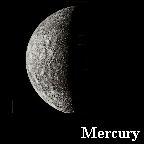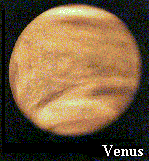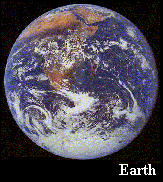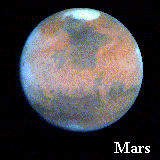The sample quiz below was formatted to show the correct answers, it is a "teaching" quiz. Showing the correct answers is optional. For a quiz to give an actual test you would format it to not show the correct answers.

Warning! Pressing [ENTER] or [RETURN] will transmit & terminate this quiz!

06-07-00
Illinois Institute of Paranormalcy, Normal Illinois
Prof. Abraham Lincoln

# Chapter 12, Test

To end of test.

SURVEY INSTRUCTIONS

These questions are not graded. They are used to gather information.

S1. Have you ever taken this course before?

1. Yes
2. No

S2. If you have taken this test before what was your score the LAST time you took it?

TEST INSTRUCTIONS

Read the text and then answer the questions that follow. Correct answers at the end of the quiz.

Questions were selected in random order by the software and then grouped together according to which document they are linked to.

The limit to document size is 32,000 characters per document with up to 20 documents per test. 999 questions per quiz

THE INNER PLANETSThe nearest planet to the Sun is Mercury. Mercury is an airless, rocky planet with no satellites, orbits the Sun at an average distance of 36 million miles, takes 88 days to complete one orbit and has a diameter of 3000 miles.Venus is the second planet. It has a thick, opaque, carbon dioxide atmosphere at an average temperature of 800 degrees centigrade. It has no satellites, orbits the Sun at an average distance of 87 million miles which takes 224 days, and has a diameter of 7700 miles.Earth is our planet. It has one satellite, the moon, orbits the Sun in 365.25 days at 93 million miles, and has a diameter of 7927 miles.Mars is the fourth planet. It has a very thin atmosphere, two small satellites, orbits the Sun in 687 days at 143 million miles and has a diameter of 42
1. Which planet has a thick carbon dioxide atmosphere?

1. Mars
2. Venus
3. Earth
4. Mercury
5. None of these2. Which planet do we live on?

1. 1st
2. 2nd
3. 3rd
4. 4th
5. 5th

3. BONUS QUESTION.

Describe a typical inner planet (as opposed to an outer planet), as far as size, atmosphere, number of moons, etc.4. What is the diameter of Venus?

Match the following....
 5 ```Asteroid A) Is rarely the farthest from the Sun ``` Answer ---> 5 C 6 ```Venus B) Diameter of 88,700 miles ``` Answer ---> 6 F 7 ```Jupiter C) Minor body circling the sun ``` Answer ---> 7 B 8 ```Mercury D) Highly elliptical orbit ``` Answer ---> 8 E 9 ```Pluto E) Airless rocky planet ``` Answer ---> 9 D 10 ```Mars F) Carbon dioxide atmosphere ``` Answer ---> 10 I 11 ```Saturn G) 1,783 million miles from the Sun ``` Answer ---> 11 H 12 ```Neptune H) Prominent rings ``` Answer ---> 12 A 13 ```Earth I) Two small satellites ``` Answer ---> 13 J 14 ```Uranus J) Only one satellite ``` Answer ---> 14 G

To start of test.

After reviewing your answers press the Transmit button to complete this test.

Test created using "Harris Test" - http://keyhut.com/indexpos.htm